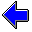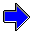Java Security Settings:
This web page employs Java, which requires specific security settings for correct operation.
If the applets on this page do not run correctly, consult the Virtual Chemistry Experiments FAQ
or the Physlet Physics web site for establishing the correct security settings.

Gas Laws

Concepts

In the previous experiments, you have examined two important gas laws. Boyle's Law states that the product of the pressure and volume of a gas is a constant for a fixed amount of gas and constant temperature. Charles's Law (sometimes called the Gay-Lussac Law) states that the volume of a gas is directly proportional to the temperature of the gas, provided the amount of gas and pressure are held constant.

In this experiment you will examine a third important gas law: Avogadro's Law. During the first half of the nineteenth century, Lorenzo Romano Amedeo Carlo Avogadro, Count of Quaregna and Cerreto, made major contributions towards elucidating reaction stoichiometry and explaining why compounds reacted in certain well-defined integer ratios. These studies led Avogadro to address the question of how the amount of gas affect the volume of the gas and how best to think about the amount of a gas. Experimentally, the easiest way to quantify the amount of gas is as a mass. Avogadro played an important role in establishing the existence of atoms. The number of molecules in a mole is named after him.

Experiment

Objectives

• Determine how the amount of gas in a fixed volume at a fixed pressure and temperature depends upon the identity of the gas.
• Determine molar volume and the molar concentration of a gas at STP.

The experimental apparatus consists of a glass bulb which will be evacuated and then filled with a gas at standard temperature and pressure (STP). STP corresponds with 1 atm pressure (760 torr) and 0 oC. The bulb will be weighed when evacuated and when filled with a gas at STP. The difference in mass is the mass of the gas itself. The volume of the bulb is 500.0 mL.

This measurement will be performed for several different gases. For each gas, experimentally determine the mass of the gas at STP and calculate the following properties.

Density
The density, d, is the ratio of the mass to the volume (in mL): d = m/V

Concentration
The concentration, C, is the ratio of the moles of gas to the volume (in L): C = n/V

The number of moles, n, is the mass of the gas divided by the formula weight, FW, of the gas: n = m/FW

The formula weight, FW, is the mass of one mole of the gas.

Molar Volume
The molar volume, Vm, is the volume of one mole of gas and is the reciprocal of the concentration: Vm = 1/C = V/n

Experimental Details

In practice, this experiment would be performed in the following fashion. The bulb would be connected to a manifold, to which is attached a tank of the gas of interest, a vacuum pump, and a manometer. If gas is being added, the valve to the vacuum pump is be closed and the valve to the gas tank is gently opened to vent gas into the system. Gas is added until the desired pressure is displayed on the manometer. Gas is removed from the bulb by closing the valve to the gas tank and gently opening the valve to the vacuum pump. It is possible to completely evacuate the bulb using the vacuum pump. After the bulb contains the desired pressure of gas, the bulb is removed from the manifold and placed on a balance. The mass of the bulb containing gas minus the mass of the evacuated bulb is the mass of gas.

The open end of the manometer is connected to the manifold; thus the pressure recorded by the manometer is the pressure in the manifold and thus the bulb, which is open to the manifold.

In this simulated experiment, the pressure and mass are measured simultaneously (even though this is not really possible in practice).

The glass bulb has a volume of 500.0 mL. The temperature of the gas is held at 0 oC. You will need to add sufficient gas to produce a pressure of exactly 760 torr (1 atm). This temperature and pressure correspond to STP.

When a new gas is selected, the bulb is evacuated to remove the old gas. Each gas is given a color to illustrate its presence in the bulb. In reality, all of the gases used in this experiment are colorless except chlorine, which has green color.

Questions
After performing the measurements with each gas, answer the following questions.
1. How does the density of the gas at STP depend upon the identity of the gas?
2. How does the molar concentration at STP depend upon the identity of the gas?
3. How does the molar volume at STP depend upon the identiy of the gas?

 Select Gas Helium Nitrogen Oxygen Neon Chlorine ArgonCharles's Law                     Ideal Gas Law and the Gas ConstantGas Laws Home PageVirtual Chemistry Home Page